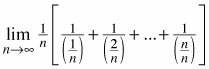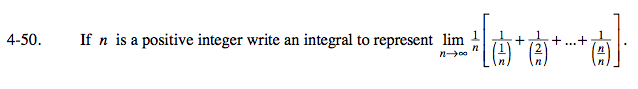### Home > CALC > Chapter 4 > Lesson 4.2.1 > Problem4-50

4-50.

If n is a positive integer write an integral to represent. Homework Help ✎Notice that this is a Riemann sum with infinitely many rectangles.

And a Riemann sum with infinitely many rectangles is the Definition of an Integral:

Can you rewrite this as an integral?

$\lim_{n\rightarrow \infty }\frac{1}{n}=\lim_{x\rightarrow 0}\Delta x=dx..............\text{ so we can substitute }\frac{1}{n}\text{ with }dx.$

The dx represents the infinitely small width of each rectangle.
Now lets find the height of each rectangle.
Heights, of course, are represented by a function, f(x).
But what is f(x)?

Since x is a variable, we will let x represent the part of the series that is changing:

$\text{This is beginning to look more like an integral: }\lim_{n\rightarrow \infty }\frac{1}{x}dx$

We still need to find the bounds of the integral.

$\text{The lowest value of }x \text{ is } \frac{1}{n}. \text{ Since }\lim_{n\rightarrow \infty }\frac{1}{n}=0,\text{ the lower bound is }0.$